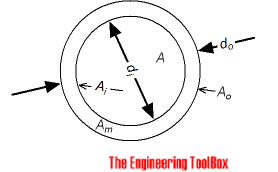Engineering ToolBox - Resources, Tools and Basic Information for Engineering and Design of Technical Applications!

# Pipe Equations

## Calculate cross-sectional areas, weight of empty pipes, weight of pipes filled with water, inside and outside surface areas.### Cross Sectional inside Pipe Area

Cross-sectional inside area of a pipe can be calculated as

Ai = π (di / 2)2

= π di 2 / 4                                 (1)

where

Ai = cross-sectional inside area of pipe (m2, in2)

di = inside diameter (m, in)

### Cross Sectional Pipe Wall Area

The cross-sectional wall area - or area of piping material - can be calculated as

Amπ (do / 2)2 - π (di / 2)2

= π (do 2 - di 2) / 4                               (2)

where

Am = cross-sectional wall area of pipe (m2, in2)

do = outside diameter (m, in)

### Weight of Empty Pipes

Weight of empty pipes per unit length can be calculated as

wpρm Am

= ρm (π (do / 2)2 - π (di / 2)2

= ρm  π (do2 - di2) / 4                              (3)

where

wp = weight of empty pipe per unit length (kg/m, lb/in)

ρs = density of pipe material (kg/m3, lb/in3)

### Weight of Liquid in Pipes

Weight of liquid in pipes per unit length can be calculated as

wl = ρl A

= ρl π (di / 2)2

= ρl π di2 / 4                                   (4)

where

wl = weight of liquid in pipe per unit length of pipe (kg, lb)

ρl = density of liquid (kg/m3, lb/in3)

### Weight of Pipe filled with Liquid

Weight of pipe filled with liquid per unit length can be calculated as

w = wl + wp                          (5)

where

w = weight of pipe and liquid per unit length of pipe (kg, lb)

### Outside Surface Area of Pipes

Outside surface area of steel pipes per unit length can be calculated as

Ao = 2 π (do / 2)

=  π do                            (6)

where

Ao = outside area of pipe - per unit length of pipe (m2, in2)

### Inside Surface Area of Pipes

Inside surface area of steel pipes per unit length can be calculated as

Ai2 π (di / 2)

=  π di                             (7)

where

Ai = inside area of pipe - per unit length of pipe (m2, in2)

## Related Topics

• Dimensions - Sizes and dimensions of pipes and tubes, and their fittings - inside and outside diameter, weight and more.

## Engineering ToolBox - SketchUp Extension - Online 3D modeling!

Add standard and customized parametric components - like flange beams, lumbers, piping, stairs and more - to your Sketchup model with the Engineering ToolBox - SketchUp Extension - enabled for use with the amazing, fun and free SketchUp Make and SketchUp Pro .Add the Engineering ToolBox extension to your SketchUp from the SketchUp Pro Sketchup Extension Warehouse!

Translate

## Privacy

We don't collect information from our users. Only emails and answers are saved in our archive. Cookies are only used in the browser to improve user experience.

Some of our calculators and applications let you save application data to your local computer. These applications will - due to browser restrictions - send data between your browser and our server. We don't save this data.

## Citation

• Engineering ToolBox, (2005). Pipe Equations. [online] Available at: https://www.engineeringtoolbox.com/pipes-equations-d_873.html [Accessed Day Mo. Year].

Modify access date.

. .

#### Scientific Online Calculator3 10